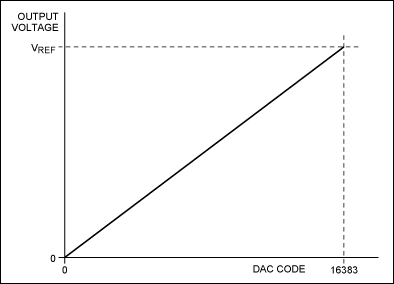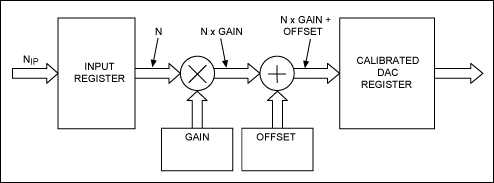# Adjusting and Calibrating Out Offset and Gain Error in a Precision DAC

### 要約

This application note defines offset and gain errors in a DAC and identifies some of the sources for that error. The article explains that the error can be calibrated out in the analog and digital domains and shows ways to accomplish it. The MAX5774 precision DAC serves as the example device.

### Overview

All DAC systems experience gain and offset error. These are analog errors caused by many factors in the DAC and in the external signal path. Gain and offset error should, therefore, be specified in the data sheet for a precision DAC.

The MAX5774 is a 32-channel, 14-bit precision DAC with gain and offset registers for each DAC channel. The MAX5774 also contains a global offset register. Using this global offset register, both device and system gain and offset errors can be calibrated out and each channel set to output a specific range.

This application note describes these DAC errors and their sources, and then describes methods for calibrating out that error in both the analog and digital domains.

### Gain and Offset Errors

An ideal 14-bit DAC has the characteristic shown in Figure 1.At code 0, the output voltage is exactly 0V, and at code 16383 the output voltage is exactly VREF. (In fact some DACs will output VREF × (2N-1/2N) at max code. However for simplicity we will assume VREF). The line is perfectly linear. If a perfectly linear DAC like this could be made at an affordable price, someone would be a millionaire.

At code 0, the output voltage is never exactly what it should be. The deviation from the ideal code 0 voltage is the offset error. Offset errors are normally bipolar and often expressed in a DAC data sheet in terms of millivolts.

The gain of a DAC is the slope of the output characteristic. Gain is generally specified in DAC data sheets in terms of %FSR (full-scale range), and measured between code zero and maximum code or, in some cases, between codes close to zero and maximum. The deviation from the ideal over the full output range is known as the gain error. Since the characteristic is never a straight line, the end points, or a point close to each end, are used in calculating gain error. If the offset error is removed from a real characteristic, then what remains at maximum code is the gain error. An exaggerated but still linear DAC characteristic is shown in Figure 2.### Integral Nonlinearity Error (INL)

This application note does not discuss how to calibrate out INL. However, INL is defined since it needs to be considered when using digital calibration.

All of the characteristics shown in Figure 2 above are perfectly linear; they are never exactly linear as shown in Figure 3 below. INL is a measure of how far the characteristic deviates from the ideal. It is measured in two ways: end to end, and best fit. To measure INL, the offset and gain errors are first removed.Most DACs are specified using the end-to-end method. The measurement is expressed in LSBs.

### Causes of Errors

Given the multiple types of DAC errors, it is virtually a "given" that the DAC's output will only be used if the signal is run through some kind of conditioning circuitry. This circuitry can range from a simple transistor or op amp to a more complex IC like the MAX15500 output conditioner. Still, it is important to realize that all stages through which the signal passes will add some amount of offset and change the gain to some degree.

In many DAC systems an external voltage reference is used to set the gain. An imperfect voltage reference will also introduce gain error.

### Calibrating Out the Errors

Systems must either be designed to perform with the required gain and offset errors without calibration, or they must be calibrated. High-accuracy systems most often require calibration, which can be done in the analog domain, the digital domain, or a combination of both.

There is an important advantage to calibrating offset and gain errors in the digital domain: most systems already have some form of digital processing in the digital domain. The process requires little, if any, hardware overhead. The disadvantage to digital calibration, however, is the introduction of ±0.5 LSB of INL (Figure 4).

The advantage of calibrating in the analog domain is that it does not incur the INL penalty. The downside is that it often requires more hardware. Digital calibration is normally implemented by either a lookup table or a mathematical function (Figure 4).First, the gain error is corrected, and then an offset to correct for the offset error is added or subtracted. The effect of this is shown in Figure 5. There is a two-fold advantage of this method vs. the lookup-table method: it is simple to implement, and it is simple to calibrate with ATE at final test. This approach is linear, but that is a disadvantage. Hence, any nonlinear effects of the DAC cannot be calibrated out. This calibration can, however, be done with a lookup table, but final test calibration is very time consuming since many more points must be calibrated and that adds cost. If ultimate linearity is required, a suitable linear DAC is a better alternative.Figure 5 shows that the original noncalibrated characteristic exhibits a gain error less than 1. So, initially the calibrated DAC code follows the input code, until the gain error results in -0.5 LSB of error. At this point one code is missed and the output jumps to +0.5 LSB of error. This can be repeated as many times as necessary to calibrate the gain error. Once this is done, a simple offset is applied to correct for the offset error. If the gain error resulted in a steeper curve, codes would be missed. The effect in INL would be the same.

### Practical Solution to the Problem

The Maxim MAX5774 is a 14-bit, 32-channel DAC with integrated gain and offset calibration registers for each DAC channel. Using its global offset register, both device and system gain and offset errors can be calibrated out and each channel set to output a specific range. The MAX5774 is just one of several parts offered by Maxim with these functions.

If the MAX5774's gain calibration registers are set to 1 and the offset calibration registers (Figure 6) are set to 0, the typical gain error will be 0.1% and the typical offset error will be 8mV. Calibrating the device results in an improved gain error of 0.05% and an offset error of an impressive 300µV.### Summary

This article has defined DAC offset and gain errors and looked at some of the sources for that error in DAC systems. Ways to calibrate out these errors in both the analog and digital domains were demonstrated. Finally, the article presented an integrated solution to this DAC calibration problem with the MAX5774.